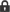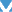Welcome to BlackGun Forum#### KennyV9Jan 13 2020 10:47 PM
•##### Alexandra.
Bn
Jan 13 2020 11:19 PM
•##### KawaKing
=))))))))))))))))))))))))))))))))))))))))))

Jan 13 2020 11:19 PM